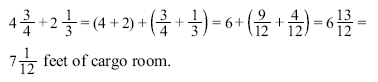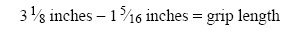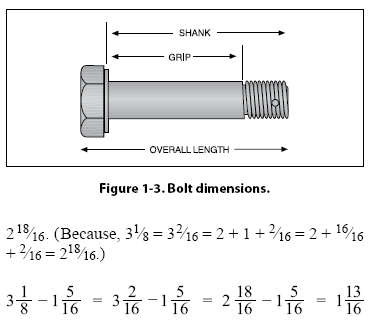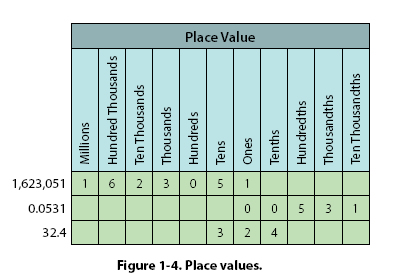CHAPTER 1. Mathematics Mixed Numbers A mixed number is a combination of a whole number and a fraction. Addition of Mixed Numbers To add mixed numbers, add the whole numbers together. Then add the fractions together by finding a common denominator. The final step is to add the sum of the whole numbers to the sum of the fractions for the final result. Example: The cargo area behind the rear seat of a small airplane can handle solids that are 43/4 feet long. If the rear seats are removed, then 21/3 feet is added to the cargo area. What is the total length of the cargo area when the rear seats are removed?Subtraction of Mixed Numbers To subtract mixed numbers, find a common denominator for the fractions. Subtract the fractions from each other (it may be necessary to borrow from the larger whole number when subtracting the fractions). Subtract the whole numbers from each other. The final step is to combine the final whole number with the final fraction. Example: What is the length of the grip of the bolt shown in Figure 1-3? The overall length of the bolt is 31/2 inches, the shank length is 31/8 inches, and the threaded portion is 15/16 inches long. To find the grip, subtract the length of the threaded portion from the length of the shank.To subtract, start with the fractions. Borrowing will be necessary because 5/16 is larger than 1/8 (or 2/16). From the whole number 3, borrow 1, which is actually 16/16. After borrowing, the first mixed number will now beTherefore, the grip length of the bolt is 113/16 inches. (Note: The value for the overall length of the bolt was given in the example, but it was not needed to solve the problem. This type of information is sometimes referred to as a “distracter" because it distracts from the information needed to solve the problem.) The Decimal Number System The Origin and Definition The number system that we use every day is called the decimal system. The prefix in the word decimal is a Latin root for the word “ten." The decimal system probably had its origin in the fact that we have ten fingers (or digits). The decimal system has ten digits: 0, 1, 2, 3, 4, 5, 6, 7, 8, and 9. It is a base 10 system and has been in use for over 5,000 years. A decimal is a number with a decimal point. For example, 0.515, .10, and 462.625 are all decimal numbers. Like whole numbers, decimal numbers also have place value. The place values are based on powers of 10, as shown in Figure 1-4.©AvStop Online Magazine                                                                                                                                                      Contact Us              Return To Books